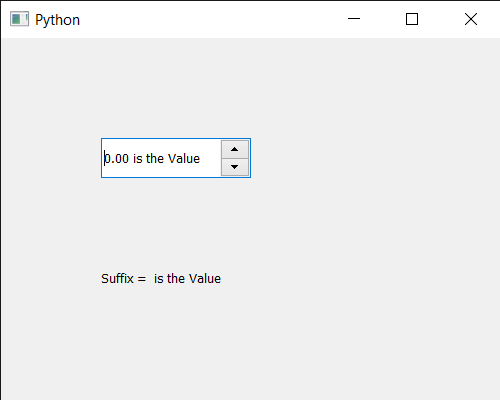Related Articles

# PyQt5 QDoubleSpinBox – Getting Suffix

• Last Updated : 14 May, 2021

In this article we will see how we can get the suffix of the QDoubleSpinBox. In linguistics, a suffix is an affix which is placed after the stem of a word. Common examples are case endings, which indicate the grammatical case of nouns or adjectives, and verb endings, which form the conjugation of verbs. An inflectional suffix is sometimes called a desinence or a grammatical suffix or ending. By default there is no suffix to the double spin box although we can add suffix to it with the help of setSuffix method.

In order to do this we will use suffix method with the double spin box object.
Syntax : dd_spin.suffix()
Argument : It takes no argument
Return : It returns string

Below is the implementation

## Python3

 `# importing libraries``from` `PyQt5.QtWidgets ``import` `*``from` `PyQt5 ``import` `QtCore, QtGui``from` `PyQt5.QtGui ``import` `*``from` `PyQt5.QtCore ``import` `*``import` `sys`  `class` `Window(QMainWindow):` `    ``def` `__init__(``self``):``        ``super``().__init__()` `        ``# setting title``        ``self``.setWindowTitle(``"Python "``)` `        ``# setting geometry``        ``self``.setGeometry(``100``, ``100``, ``500``, ``400``)` `        ``# calling method``        ``self``.UiComponents()` `        ``# showing all the widgets``        ``self``.show()` `    ``# method for components``    ``def` `UiComponents(``self``):` `        ``# creating double spin box``        ``d_spin ``=` `QDoubleSpinBox(``self``)` `        ``# setting geometry to the double spin box``        ``d_spin.setGeometry(``100``, ``100``, ``150``, ``40``)` `        ``# setting suffix``        ``d_spin.setSuffix(``" is the Value"``)` `        ``# step type``        ``step_type ``=` `QAbstractSpinBox.AdaptiveDecimalStepType` `        ``# adaptive step type``        ``d_spin.setStepType(step_type)` `        ``# creating a label``        ``label ``=` `QLabel(``"GeeksforGeeks"``, ``self``)` `        ``# setting geometry to the label``        ``label.setGeometry(``100``, ``200``, ``300``, ``80``)` `        ``# making label multi line``        ``label.setWordWrap(``True``)` `        ``# getting suffix``        ``value ``=` `d_spin.suffix()` `        ``# setting text to the label``        ``label.setText(``"Suffix = "` `+` `str``(value))`      `# create pyqt5 app``App ``=` `QApplication(sys.argv)` `# create the instance of our Window``window ``=` `Window()` `# start the app``sys.exit(App.``exec``())`

Output :Attention geek! Strengthen your foundations with the Python Programming Foundation Course and learn the basics.

To begin with, your interview preparations Enhance your Data Structures concepts with the Python DS Course. And to begin with your Machine Learning Journey, join the Machine Learning – Basic Level Course

My Personal Notes arrow_drop_up